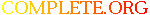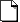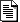Complete.Org: Mailing Lists: Archives: freeciv-ai: November 2002: [freeciv-ai] Measure the AI ability to harvest resources# [freeciv-ai] Measure the AI ability to harvest resources

[Top] [All Lists]

 To: freeciv-ai@xxxxxxxxxxx Subject: [freeciv-ai] Measure the AI ability to harvest resources From: Raimar Falke Date: Sun, 24 Nov 2002 22:53:13 +0100

```I'm currently updating my unit agents patch. To measure the
performance I'm using the attached patch. It measures the resources
which a player collected with all his cities. Leading "2:" is from
freelog. Than turn and than playername. Next 3 numbers are the food,
shield and trade collected to far. Next 3 luxury, tax and science. s
is the food*FOOD_WEIGHTING + shield*SHIELD_WEIGHTING +

Results are for the attached savegame (snapshot after 30 turns,
afterwards the agents got "unstable"):

all 4 player are controlled by server-AI:

2: 29:            Wang Gon:(f s t)=(  75  125  138) (l t s)=(   0   11  127) s=
5206 uf= 429
2: 29:      Guntis Ulmanis:(f s t)=( 113  147  146) (l t s)=(   0    1  145) s=
6398 uf= 440
2: 29:   Richard Lionheart:(f s t)=( 112  189   59) (l t s)=(   0   25   34) s=
6049 uf= 499
2: 29:             Genghis:(f s t)=( 105   60  144) (l t s)=(   0    0  144) s=
4743 uf= 408

Agents (CMA, SMA and EA) control Genghis (the player with the lowest rating):
2: 29:            Wang Gon:(f s t)=(  75  125  138) (l t s)=(   0   11  127) s=
5206 uf= 441
2: 29:      Guntis Ulmanis:(f s t)=( 113  147  146) (l t s)=(   0    1  145) s=
6398 uf= 443
2: 29:   Richard Lionheart:(f s t)=( 112  189   59) (l t s)=(   0   25   34) s=
6049 uf= 493
2: 29:             Genghis:(f s t)=(  87  187   90) (l t s)=(   0   13   77) s=
5912 uf= 438
+25%

agents control Guntis (highest rating):
2: 29:            Wang Gon:(f s t)=(  75  125  138) (l t s)=(   0   11  127) s=
5206 uf= 445
2: 29:      Guntis Ulmanis:(f s t)=( 125  223  105) (l t s)=(   0   28   77) s=
7426 uf= 423
2: 29:   Richard Lionheart:(f s t)=( 112  189   59) (l t s)=(   0   25   34) s=
6049 uf= 493
2: 29:             Genghis:(f s t)=( 105   60  144) (l t s)=(   0    0  144) s=
4743 uf= 413
+16%

So the agents are better than a hard server-AI. Even so it looks like
Genghis is penalized by his starting position.

To have a fairer ground for comparing (and also to see how bad/good my
EA is) I now show all the players the map at the start of the game.

2: 29:            Wang Gon:(f s t)=(  71  147  150) (l t s)=(   0   42  108) s=
5648 uf=4000
2: 29:      Guntis Ulmanis:(f s t)=( 123  160  162) (l t s)=(   0   11  151) s=
7001 uf=4000
2: 29:   Richard Lionheart:(f s t)=( 108  195   92) (l t s)=(   0   30   62) s=
6471 uf=4000
2: 29:             Genghis:(f s t)=( 145  173  139) (l t s)=(   0   19  120) s=
7364 uf=4000

All AIs perform better. Genghis is +55%. I thought that the AI is omniscience.

agents control Genghis (now the highest player):
2: 29:            Wang Gon:(f s t)=(  71  147  150) (l t s)=(   0   42  108) s=
5648 uf=4000
2: 29:      Guntis Ulmanis:(f s t)=( 123  160  162) (l t s)=(   0   11  151) s=
7001 uf=4000
2: 29:   Richard Lionheart:(f s t)=( 108  195   92) (l t s)=(   0   30   62) s=
6471 uf=4000
2: 29:             Genghis:(f s t)=( 163  246  173) (l t s)=(   0   66  107) s=
9355 uf=4000
+25%

agents control Wang:
2: 29:            Wang Gon:(f s t)=( 107  188  195) (l t s)=(   0   76  119) s=
7569 uf=4000
2: 29:      Guntis Ulmanis:(f s t)=( 123  160  162) (l t s)=(   0   11  151) s=
7001 uf=4000
2: 29:   Richard Lionheart:(f s t)=( 108  195   92) (l t s)=(   0   30   62) s=
6471 uf=4000
2: 29:             Genghis:(f s t)=( 145  173  139) (l t s)=(   0   19  120) s=
7364 uf=4000
+33%

I haven't searched why the server AIs perform badly. It is quite
possible that they built warriors or barracks. Nevertheless this is an
error. Everybody should built cities the first dozen rounds. And
explore huts (no huts here to be more fair).

Other findings:
- the agents perform better if the time horizon is bigger (tested for
12, 24 and 50 turns)
- the agents perform better if the EA is greedy (discovers any many
tiles as possible, independent of the distance to a city)

Raimar

--
email: rf13@xxxxxxxxxxxxxxxxx
"We've all heard that a million monkeys banging on a million typewriters
will eventually reproduce the entire works of Shakespeare.
Now, thanks to the Internet, we know this is not true."
```start-ai.gz
Description: application/gunzipmeasure_ai1.diff
Description: Text documentsettings
Description: Text document